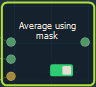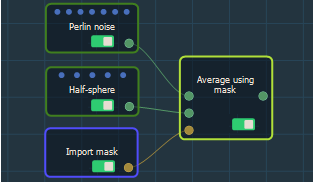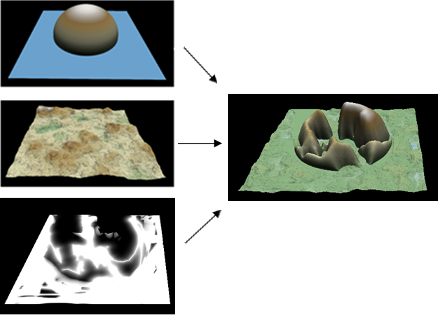This node calculates the average between two terrains at the areas defined by a mask.

To add a node, right click in the Graph Editor and select Create Node  > Terrain Composition > Average using mask.At each vertex, if the value of the mask is equal to 1 (white color), the height of the output terrain is equal to the height of the first input terrains. The second input terrain is therefore ignored for this vertex.
If the value of the mask is equal to 0 (black color), the height of the output terrain is equal to the height of the second input terrains. The first input terrain is therefore ignored for this vertex.
If the value of the mask is between 0 and 1, the height of the second input terrain is multiplied by the subtraction of 1 and the value of the mask before being added to the height of the first input terrain multiplied by the value of the mask.

For example:Both input terrains and the input mask can have different sizes and resolutions. The size and resolution of the output terrain are determined by those of the first terrain, and the second terrain and the mask are enlarged or reduced so that their size corresponds exactly to the size of the output terrain.